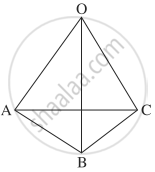# If in a tetrahedron, edges in each of the two pairs of opposite edges are perpendicular, then show that the edges in the third pair is also perpendicular. - Mathematics and Statistics

Sum

If in a tetrahedron, edges in each of the two pairs of opposite edges are perpendicular, then show that the edges in the third pair is also perpendicular.

#### SolutionLet O-ABC be a tetrahedron. Then (OA, BC), (OB, CA) and (OC, AB) are the pair of opposite edges.

Take O as the origin of reference and let bar"a", bar"b" and bar"c" be the position vectors of the vertices A, B and C respectively. Then

bar"OA" = bar"a",  bar"OB" = bar"b",  bar"OC" = bar"c",

bar"AB" = bar"b" - bar"a", bar"BC" = bar"c" - bar"b" and bar"CA" = bar"a" - bar"c"

Now, suppose the pairs (OA, BC) and (OB, CA) are perpendicular to each other.

Then bar"OA". bar"BC" = 0, i.e. bar"a".(bar"c" - bar"b") = 0

∴ bar"a" .bar"c" - bar"a" . bar"b" = 0      ....(1)

and bar"OB".bar"CA" = 0, i.e. bar"b".(bar"a" - bar"c") = 0

∴ bar"b".bar"a" - bar"b".bar"c" = 0

∴ bar"a".bar"b" - bar"b".bar"c" = 0     ...(2)

Adding (1) and (2), we get

bar"a".bar"c" - bar"b".bar"c" = 0

∴ bar"c".bar"b" - bar"c".bar"a" = 0

i.e. bar"c".(bar"b" - bar"a") = 0

∴  bar"OC" . bar"AB" = 0

∴  the third pair (OC, AB) is perpendicular.

Concept: Representation of Vector
Is there an error in this question or solution?